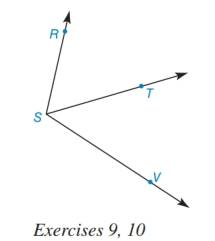Chapter 1.5, Problem 10E### Elementary Geometry for College St...

6th Edition
Daniel C. Alexander + 1 other
ISBN: 9781285195698

#### Solutions

Chapter
Section### Elementary Geometry for College St...

6th Edition
Daniel C. Alexander + 1 other
ISBN: 9781285195698
Textbook Problem
1 views

# In Exercises 7 10, state the property or definition that justifies the conclusion (the “then” clause).Given that m ∠ R S T = m ∠ T S V , then S T → bisects ∠ R S V .To determine

To find:

The property or definition that justifies the conclusion of the statement “Given that mRST=mTSV, then ST bisects RSV.”

Explanation

Given:

The given diagram is,

The statement is “Given that mRST=mTSV, then ST bisects RSV.”

Approach:

The given statement is based on hypothesis and conclusion. Let mRST=mTSV be the hypothesis and ST bisects RSV be the conclusion

### Still sussing out bartleby?

Check out a sample textbook solution.

See a sample solution

#### The Solution to Your Study Problems

Bartleby provides explanations to thousands of textbook problems written by our experts, many with advanced degrees!

Get Started

#### Find more solutions based on key concepts